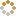﻿ Ningbo University of Technology Online Judge ::  Digital Roots
• ###  Digital Roots

• 时间限制: 1000 ms　内存限制: 65535 K
• 问题描述
• The digital root of a positive integer is found by summing the digits of the integer. If the resulting value is a single digit then that digit is the digital root. If the resulting value contains two or more digits, those digits are summed and the process is repeated. This is continued as long as necessary to obtain a single digit.

For example, consider the positive integer 24. Adding the 2 and the 4 yields a value of 6. Since 6 is a single digit, 6 is the digital root of 24. Now consider the positive integer 39. Adding the 3 and the 9 yields 12. Since 12 is not a single digit, the process must be repeated. Adding the 1 and the 2 yeilds 3, a single digit and also the digital root of 39.
• 输入
• The input file will contain a list of positive integers, one per line. The end of the input will be indicated by an integer value of zero.
• 输出
• For each integer in the input, output its digital root on a separate line of the output.
• 样例输入
• ```24
39
0
```
• 样例输出
• ```6
3
```
• 提示
• `无`
• 来源
• ```Greater New York 2000
HDOJ-1013```
• 操作
﻿